Perpendicular and Parallel

Perpendicular

Two lines are perpendicular to each other if they form a $90^\circ$ degree angle with each other. We can also say that they are at right angles to each other. We use a little box in the corner of the angle to indicate that it is a right angle, as shown in the picture below.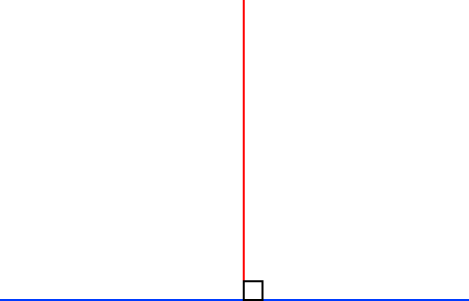Parallel

Two lines are parallel if they never touch or cross, and always head off in the same direction. Parallel lines can never cross because they are always the same distance apart.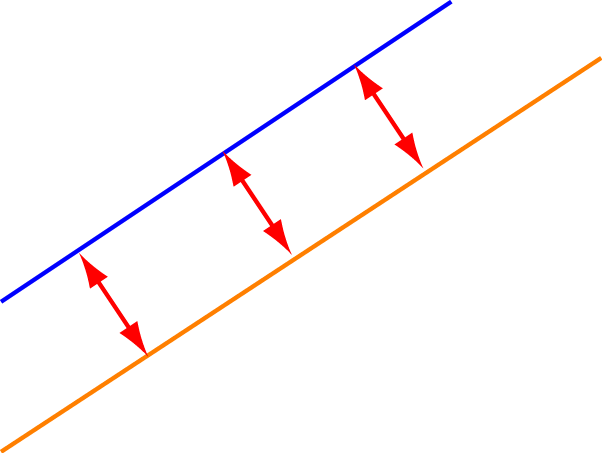The orange and blue lines in both of the examples below are parallel: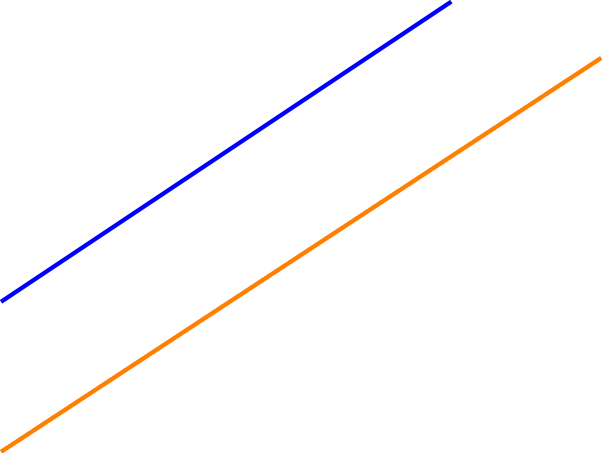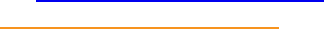You can turn a pair of parallel lines into a pair of perpendicular lines by rotating one of them through $90^\circ$. Surprisingly enough, you can also turn a pair of perpendicular lines into a pair of parallel lines by rotating one of them through $90^\circ$.

Other Things can be Parallel!

Curves and surfaces can also be parallel. It just means that they are always the same distance apart and never touch each other. Here are some examples: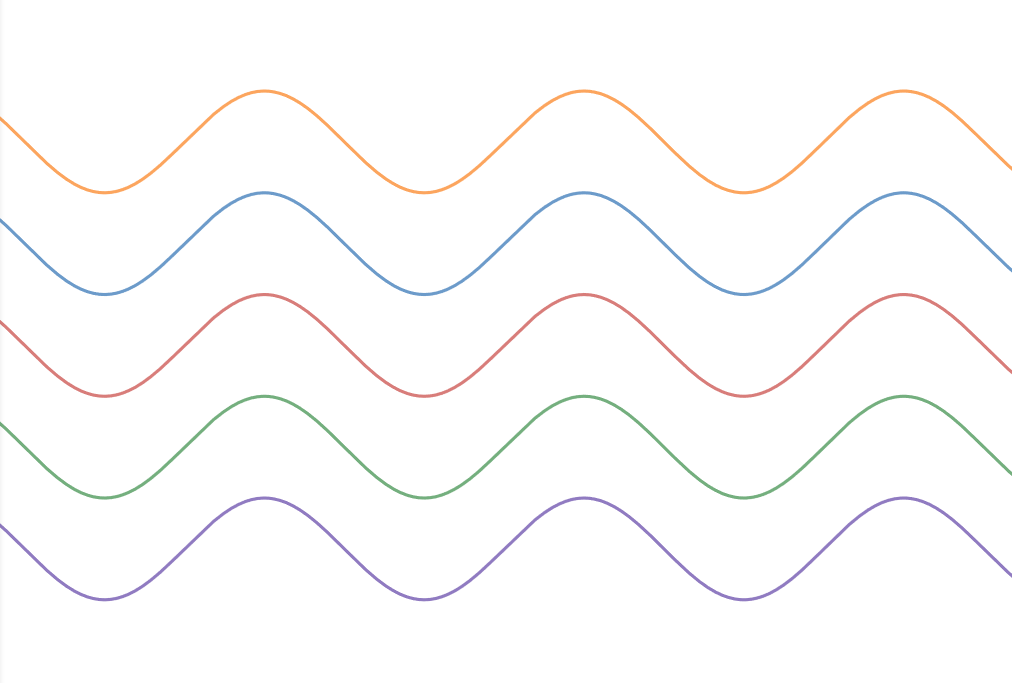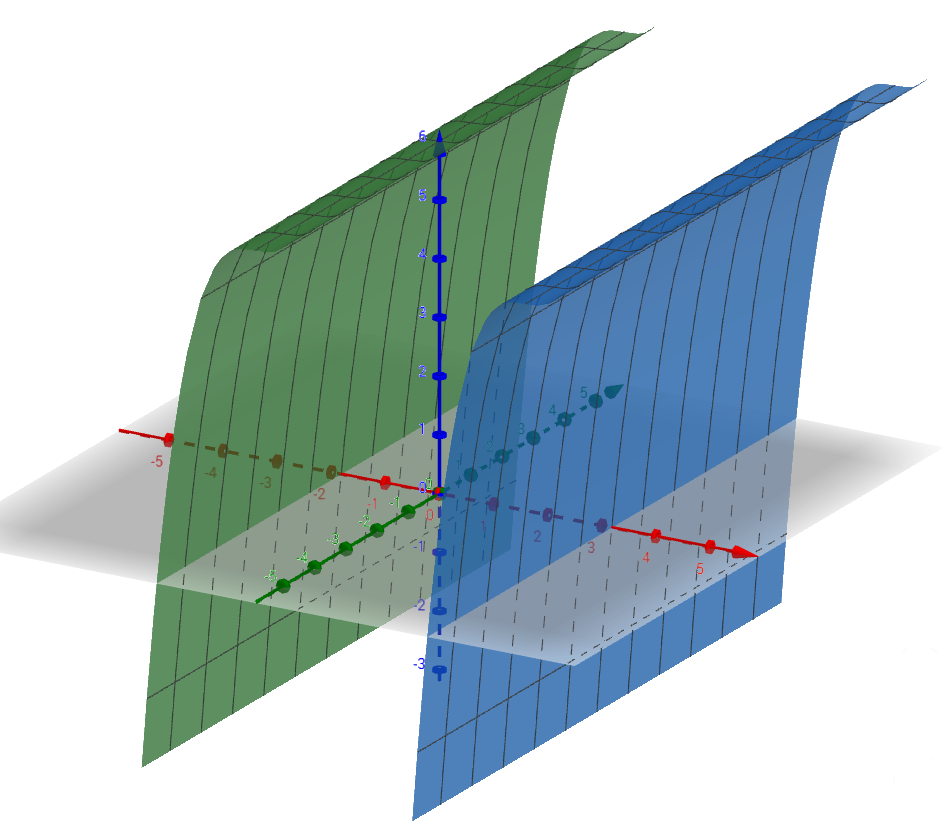Planes can also be parallel: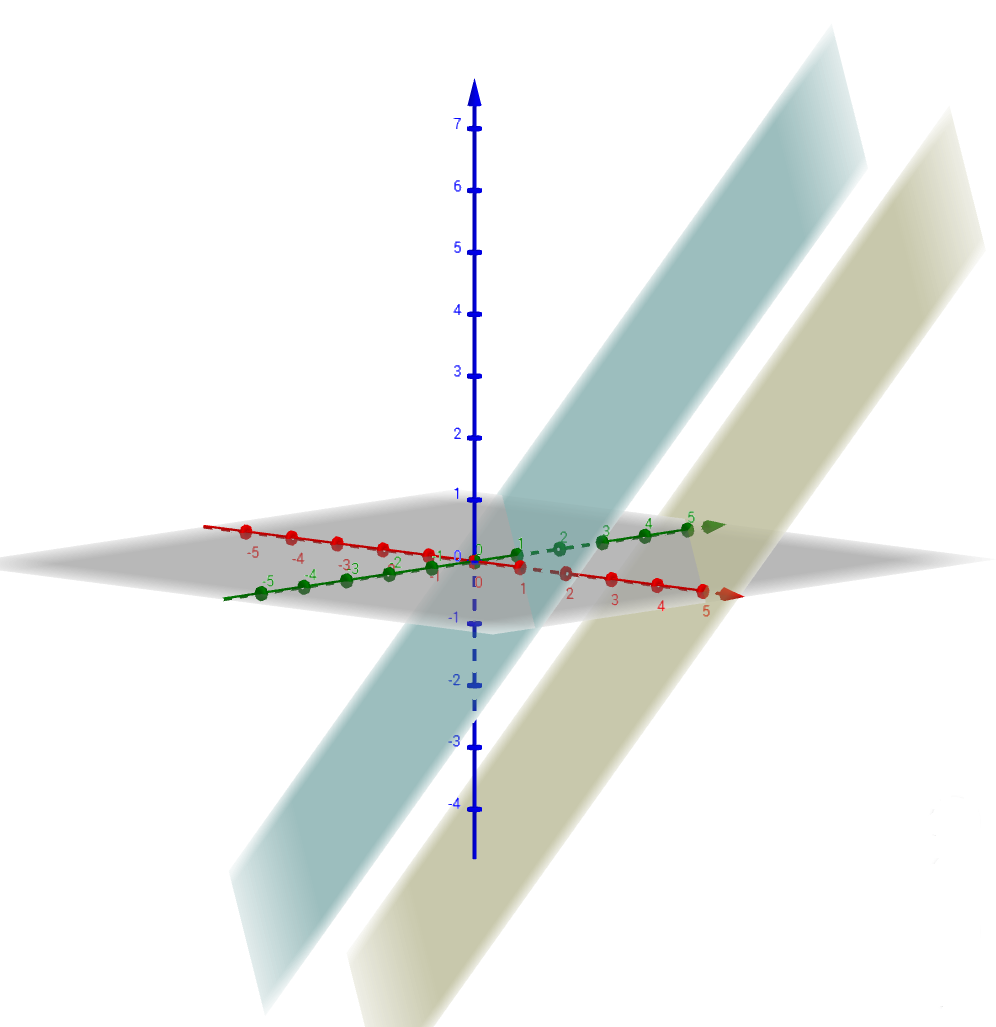Lines can be parallel to planes, and lines can be perpendicular to planes as well. We won't think about that here - that's a more advanced topic.

Description

In this mini book, you will learn about

1. Alternate angles
2. Cointerior angles
4. Vertically Opposite Angles

and several other topics related to lines and angles.

Audience

Year 10 or higher

Learning Objectives

To learn the basics of Lines and Angles stream of Geometry

Author: Subject Coach